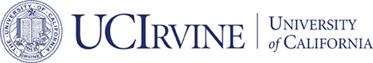# Published## Nonconforming Virtual Element Method for 2m-th Order Partial Differential Equations in R^n

### Long Chen and Xuehai Huang

#### Mathematics of Computation

ABSTRACT:

A uniform construction of the $H^m$-nonconforming virtual
elements of any order $k$ is developed on any shape of polytope in
$\mathbb R^n$ with constraints $m\leq n$ and $k\geq m$.  As a vital
tool in the construction, a generalized Green's identity for $H^m$
inner product is derived.  The $H^m$-nonconforming virtual element
methods are then used to solve the $m$-harmonic equation.  After
establishing a bound on the jump related to the weak continuity, the
optimal error estimate of the canonical interpolation, and the norm
equivalence of the stabilization term, the optimal error estimates are
derived for the $H^m$-nonconforming virtual element methods.